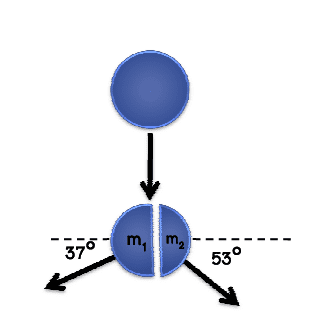# Momentum + Explosion

## Homework Statement

A grenade is falling from the height 19.6m .Finding velocity of both masses(fragments), if m1 is equal to 2 times of m2## Homework Equations

Conservation of Momentum
v2 = u2 + 2as

## The Attempt at a Solution

I think this is a pure mathematic problem :/ I can cut the mass out of my equation but I stuck with the velocities of the both masses

So, what you need to do is to resolve the m1 and m2 into both x & y component. After that, solve the system by applying the momentum conservation principle at both X and Y component.

Last edited by a moderator:
So, what you need to do is to resolve the m1 and m2 into both x & y component. After that, solve the system by applying the momentum conservation principle at both X and Y component.
I got
m1 anout 35
lol

Last edited by a moderator:
Merlin3189
Homework Helper
Gold Member
... I found the velocity of m1 is -30.2ms-1 and m2 is 50.3 ms-1 respectively....
Looking at the horizontal components,
m1 x v1 cos37 should equal 2m1 x v2 cos 53
m1 x 30.2 cos37 = 24.12 m1 and 2m1 x 50.3 cos 53 =60.54 m1 which does not match.

I got m2 about 47 m1 anout 35
I take it, these are the velocities of those masses, so v2 about 47 v1 anout 35, so horizontal components of momentum,
m1 x 35 cos37 = 28 m1 and 2m1 x 47 cos 53 = 57 m1

Perhaps we can see your full working, then have more chance of spotting errors. My own arithmetic juggling is not that good and I had to rework it a couple of times when my checks did not come right. (I managed to swap v1 and v2 on my way through the calculation!)

BTW for the final answer I assume the grenade explodes after it has fallen 19.6m. The question is a bit vague. Maybe it is dropped from 19.6m but explodes halfway down! On the other hand, if it is falling from 19.6m and explodes after it has fallen 19.6m, then it is on the ground and I think that would make a difference.

Edit: removed a comment about the sign of the velocity, which was not helpful.